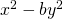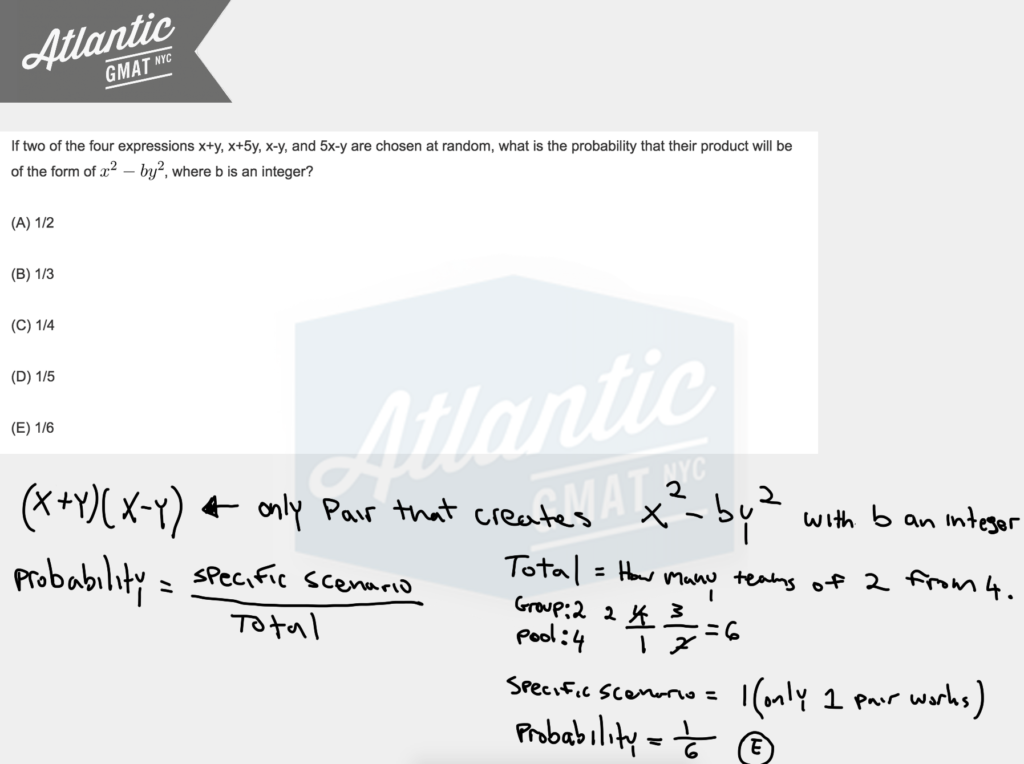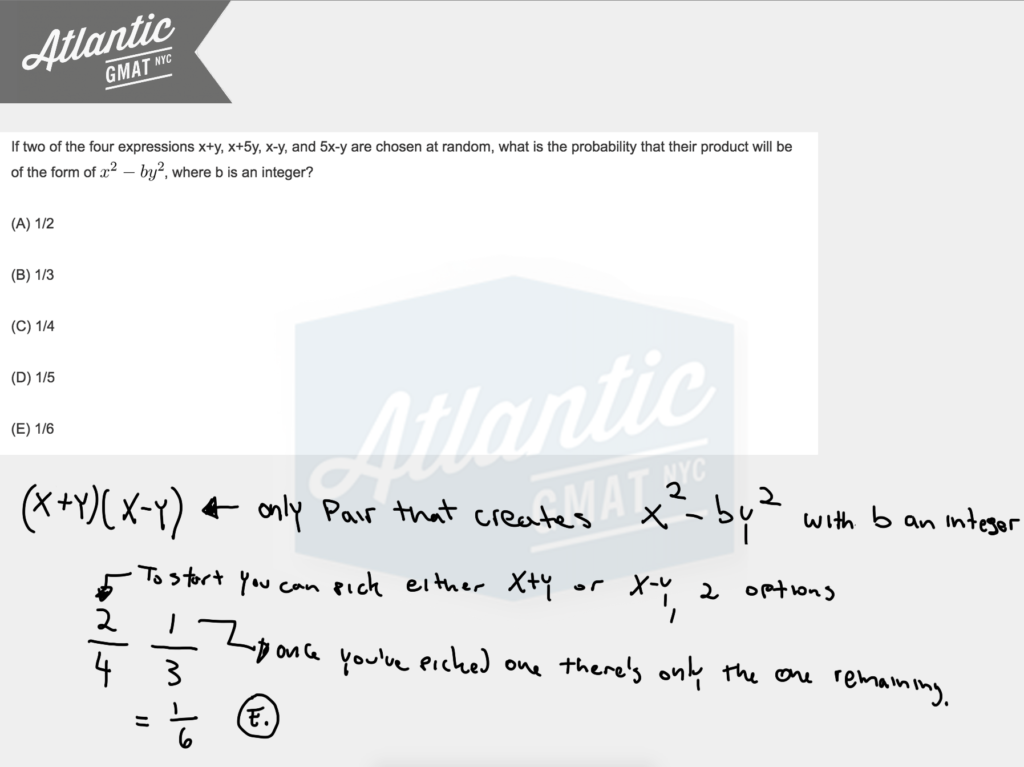If two of the four expressions x+y, x+5y, x-y, and 5x-y are chosen at random, what is the probability that their product will be of the form of, where b is an integer?

(A) 1/2

(B) 1/3

(C) 1/4

(D) 1/5

(E) 1/6

Be super comfortable with difference of squares. You're almost guaranteed to need it on your GMAT. You need to know it forwards, backwards, and upside-down. There isn't much content you need to know for the GMAT quant but in order to be consistently successful so that you can go in there on test day and snag your 700+ score you need complete fluency with that limited content. On this one we're looking for a product of two terms that will produce difference of squares. So we need something in the format: (x+y(x-y). There's only one pairing that gives us the right format. Now you have to figure out what that means in terms of probability. In general, probability is specific scenario/total scenarios. You can calculate the total and the specific scenarios using the slot method. How many teams of 2 can you make from four things? (4*3)/(1*2) = 6. So that's your denominator. And then there's only one team that works, the pairing of (x + y)(x - y). So that leaves you with 1/6. E. Detailed diagrams below and an in depth video explantation here: If two of the four expressionsYou also can think about this in terms of straight probability and say: 2 choices our of 4 and then 1 choice out of 3. 2/4 * 1/3 = 2/12 = 1/6. Comment with any questions or additions. Happy studies!# CONTACT

Atlantic GMAT

405 East 51st St.

NY, NY 10022

(347) 669-3545

info@AtlanticGMAT.com